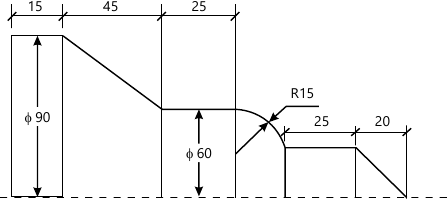MU Mechanical Engineering (Semester 7)
December 2013
Total marks: --
Total time: --
INSTRUCTIONS
(1) Assume appropriate data and state your reasons
(2) Marks are given to the right of every question
(3) Draw neat diagrams wherever necessary

1 (a) Explain in brief the importance of concurrent Engineering in product development cycle?
5 M
1 (b) List benefits of Group technology
5 M
1 (c) Write a program in C++ for 3D geometric transformations which include functions for the following
(i) Rotation @ x axis. (ii) Shearing
10 M

2 (a) Describe hardware configuration of ClM. with the help of a neat sketch.
10 M
2 (b) Specify the three principal classifications of the geometric modelling systems and write in brief about each of them
10 M

3 (a) Compare between Bezier and B-spline curve with reference to number of control points, order of continuity and surface normal.9 M
3 (b) Figure 1 shows the final profile to be generated on a MS bar stock of diameter 90mm and 150mm length by using a CNC turning center. Write CNC part program and show the toolpath.
11 M

4 (a) Figure 1 shows a component to be contour furnished on matching centre. Write the efficient APT program.
10 M
4 (b) Reflect the triangle ABC about the line 3x-4y+8 = 0. The position vector of the Co-ordinate ABC is given as A= (4, 1), B = (5. 2), C = (4. 3).
Find the composite transformation matrix and its new coordinates
10 M

5 (a) Explain any one hidden surface removal algorithm
10 M
5 (b) Compare Retrieval type and generatic type of procem planning systems
10 M

6 (a) List the different types of AS/RS and explain in brief the components of it.
12 M
6 (b) Explain the following :
(i) GKS Graphic Standard.
(ii) Co-ordinate measuring machine.
8 M

Write short notes on following with sketch / block diagram wherever possible
7 (a) Virtual Prototyping.
5 M
7 (b) Future CIM systems
5 M
7 (c) Tool length compensation
5 M
7 (d) AI in CAPP systems.
5 M
7 (e) Ray Tracing
5 M

More question papers from CAD / CAM / CIM